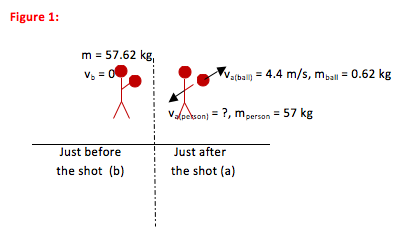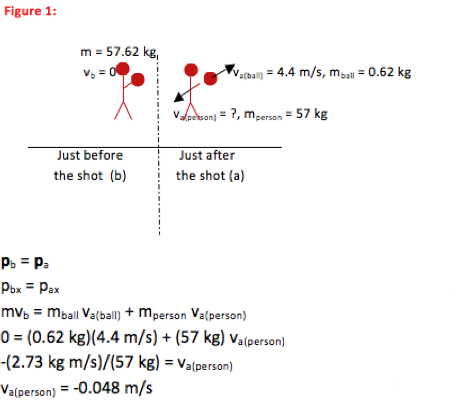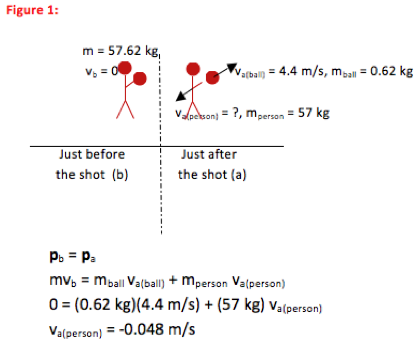# University of Wisconsin Green Bay

A 57 kg basketball player jumps vertically upward to make a shot. At the very top of her jump, she throws the basketball with a speed of 4.4 m/s. What is her recoil velocity immediately after she releases the ball? The mass of the basketball is 0.62 kg.

• In this problem, you are asked to find the recoil velocity of a basketball player after the ball is thrown. Any time you are asked to find speed or velocity of an object just before or just after a collision or separation, you should check to see if you can use Conservation of Momentum to solve the problem.

In this case, you are asked only for recoil velocity and so you know you don’t need to include effects of gravity or other external forces on the player. You can, indeed, use Conservation of Momentum.

•For Conservation of Momentum problems, you always draw a picture of the system immediately before the collision or separation and another picture immediately after. Because momentum depends on mass and velocity, label all mass and velocity information

•Pb = Pa

Any time you understand the motion of a system for which Fextermal Δt≈0, you begin with the Conservation of Momentum equation.

•The basketball player’s recoil velocity is 0.048 m/s opposite to the direction at which the ball was shot. No further mathematical solution is needed for this problem.

•In this problem, you are asked to find recoil velocity of a basketball player as she shoots a ball. This is a separation problem—a larger object separates into two pieces—and so you should first consider solving it using Conservation of Momentum. Because the problem only asked for recoil velocity (the effect of the force of the throw,) you are able to ignore any effect of external forces and so conditions to use Conservation of Momentum are, indeed, met.

Physically, you should expect the recoil velocity of the person to be small. Her mass is approximately 100 times that of the ball, and so if the magnitude of momentum for each is to be equal, her velocity should be about 1/100th that of the ball.